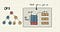# What does it even mean to traverse a tree?

## Preorder traversal

`Until all nodes are traversedStep 1 − Visit the root nodeStep 2 − Recursively traverse left subtreeStep 3 − Recursively traverse the right subtree.`

## Inorder traversal

`Until all nodes are traversed Step 1 − Recursively traverse left subtreeStep 2 − Visit the root nodeStep 3 − Recursively traverse the right subtree.`

## Postorder traversal

`Until all nodes are traversed Step 1 − Recursively traverse left subtree.Step 2 − Recursively traverse the right subtree.Step 3 − Visit the root node.`

# Depth-First Search (DFS)The depth-first search is like walking through a corn maze. You explore one path, hit a dead end, and go back and try a different one.

## DFS in Python

`graph = {    'A' : ['B','C'],    'B' : ['D', 'E'],    'C' : [],    'D' : [],    'E' : []}`
`def dfs(visited, graph, node):    if node not in visited:        print (node)        visited.add(node)        for neighbor in graph[node]:            dfs(visited, graph, neighbor)`

# BFS in Python

`graph = {  'A' : ['B','C'],  'B' : ['D', 'E'],  'C' : [],  'D' : [],  'E' : []}`
`def bfs(visited, graph, node):    visited.append(node)    queue.append(node)        while queue:        s = queue.pop(0)         print (s, end = " ")                 for neighbor in graph[s]:            if neighbor not in visited:                visited.append(neighbor)                queue.append(neighbor)`

Written by